FAIL (the browser should render some flash content, not this).AP Stats Chapter 1:
PPT

1.1
1.2
Collecting and Displaying Data

In this Unit, students will learn to:

• Understand the challenges of collecting good data
• Make inferences from analyzing data
• Understand which graphs are most suitable for certain data
• Find Measures of central tendencies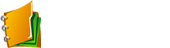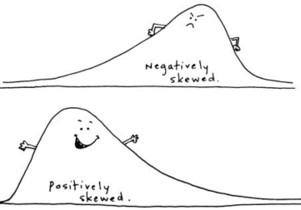AP Statistics Chapter 2:

PPT

2.1
2.2
Normal Distributions and Z-scores

In this Unit, students will learn to:

• Compute the measures of relative standning of individuals in a normal distribution
• Standardizing z-scores and percentiles
• Understand the Empirical rule of 68-95-99.7
• Use technology to find the proportion of a distribution that is between an interval of two values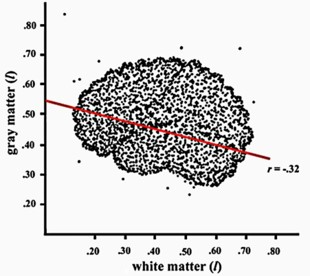AP Statistics Chapter 3:
PPT

3.1
3.2
3.3
• C3R

Linear Relationships

In this chapter, students will learn to:

Construct and interpret scatterplots for bivariate data

• Compute the correlation between two variables and describe the purpose of correlation
• Understand the purpose of a LSRLAP Statistics Chapter 4:
PPT

4.1
4.2
4.3
• C4R
Relationships Between Two Variables

In this chapter, students will learn to:

• Identify the purpose of a logarithmic transformation and how to achieve linearity
• Use two way tables and marginalization
• Identify the requirements to establish causation when experiments are not feasible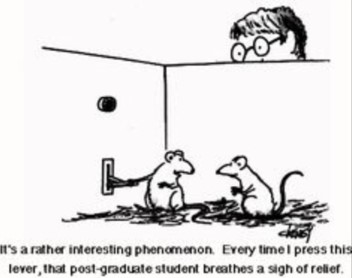AP Statistics Chapter 5:

PPT

5.1
5.2
5.3
• C5R

Data Collection

In this chapter, students will learn to:

• Distinguish and identify the characteristics of observational studies vs experiments
• Identify different sampling methods and the advantages of each
• Identify bias

• Distinguish between the purposes of randomization and blocking in an experimental designAP Statistics Chapter 6:
PPT

6.1
6.2
6.3
• C6R
Probability Rules

In this chapter, students will learn:

• Design and perform probability simulations
• Define probability concepts: Independent vs Dependent events, Joint events, union, complement events
• Solve probability problems with Bayes's rule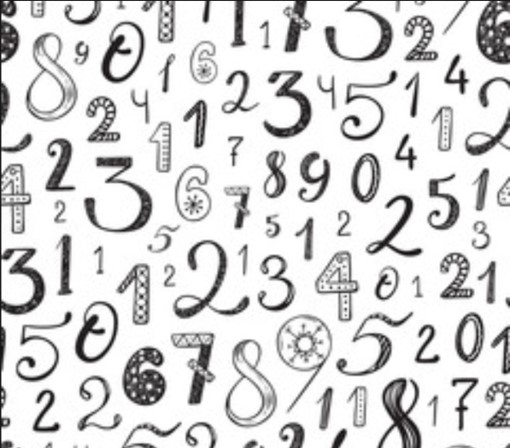AP Statistics Chapter 7:
PPT

7.1
7.2
• C7R

Random Variables

In this chapter, students will learn to:

• Distinguish between a discrete vs random variable
• Explain what a probability distribution for a random variable is

• Calculate the mean and variance of a discrete random variable
• Calculate the mean and variance of distributions formed by combining two random variablesAP Statistics Chapter 8:
PPT

8.1
8.2
• C8R

Binomial and Geometric Distributions

In this chapter, students will learn to:

• Explain what a Binomial Distribution is
• Calculate the mean and variance of a binomial random variable
• Solve problems involving geometric settings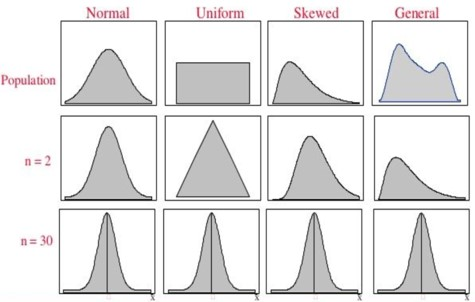AP Statistics Chapter 9:

PPT

• 9.1
• 9.2
• 9.3

Sampling Distributions

In this chapter, students will learn to:

• Define Sampling Distribution, Contrast bias and variability
• Describe the sampling distribution of a sample proportion

• Describe the Sampling distribution of a sample mean
• State Central Limit Theorem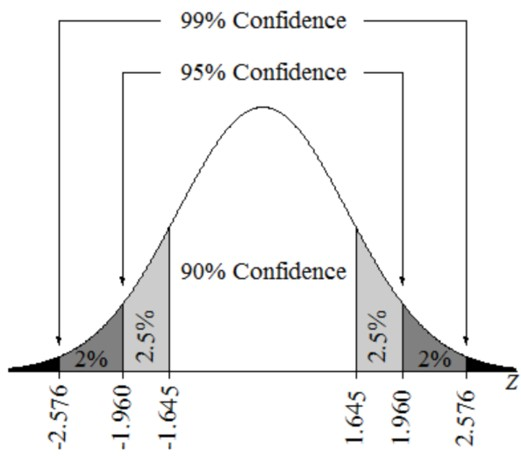AP Statistics Chapter 10:

PPT

• 10.1
• 10.2
• 10.3
Confidence Intervals

In this chapter, students will learn:

• Describe statistcal inference & margin of error
• Describe and construct a confidence interval for a population mean and for a population proportion
• Distinguish the difference between t-distribution and Normal distribution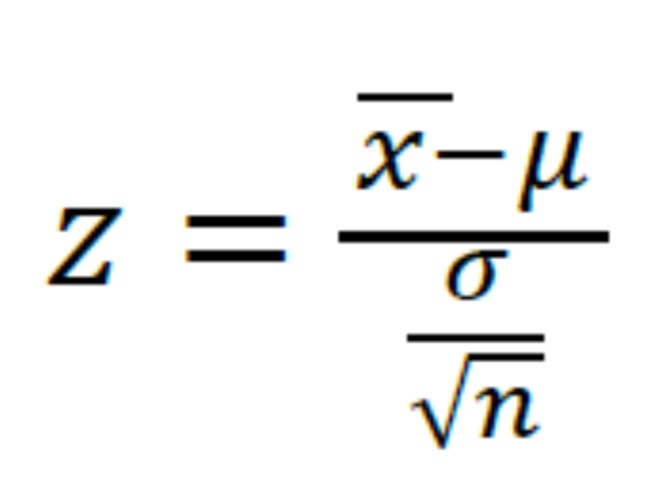AP Statistics Chapter 11:
PPT

• 11.1
• 11.2
• 11.3
• 11.4
Testing Claims

In this chapter, students will learn:

• to solve problems and justify the solution strategy using circle properties
• to explore certain circle properties involving angles, chords, and tangent lines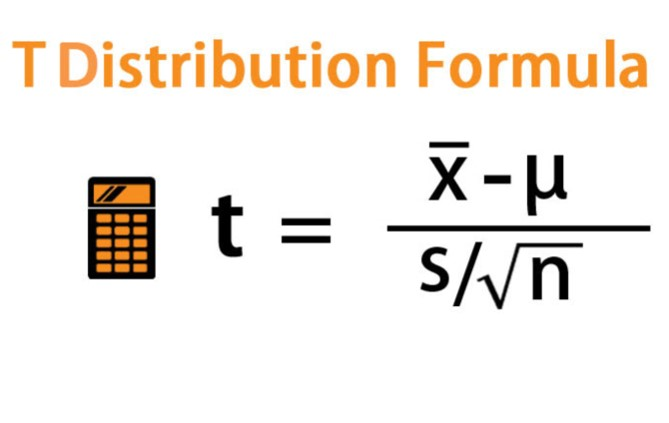AP Statistics Chapter 12:

PPT

• 12.1
• 12.2

Significance Tests

In this chapter, students will learn:

• Conduct a signficance test by mean and proportion
• Determine when to use a One Tail vs Two Tail Hypotehsis test
• Learn to calculate a the Probability of a Type I and Type II eorro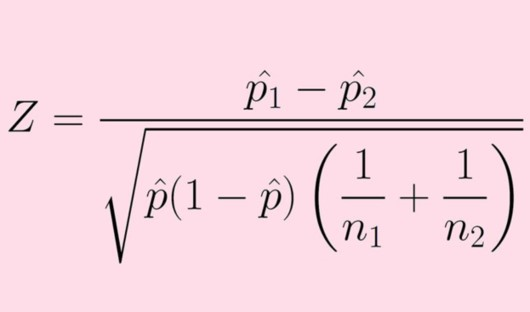AP Statistics Chapter 13:
PPT

• 13.1
• 13.2
• 13R
Comparing Two Population Parameters

In this chapter, students will learn:

• Conduct a signficance test comparing two population means and two population proportions
• You will also learn to conduct these tests using a T-distribution, where the sample population is not given.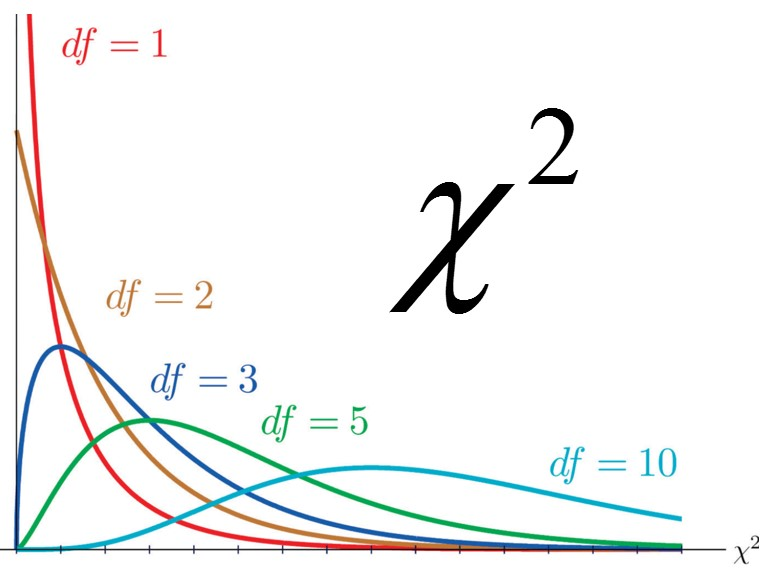AP Statistics Chapter 14:
PPT

• 14.1
• 14.2
• 14R
Chi-Squares

In this chapter, students will learn:
• to develop a project plan that involves collecting, displaying , and analysing data
• to select a sample from a population
• to understand the various aspects of collecting data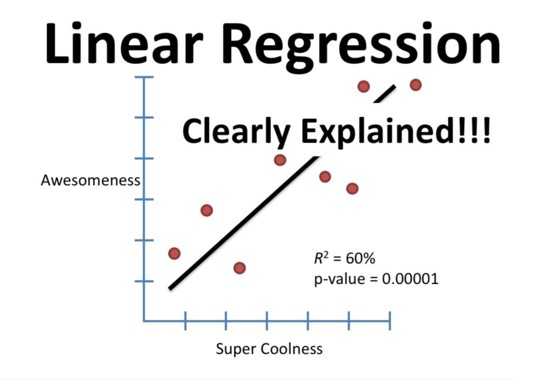AP Statistics Chapter 15:
PPT

• 15.1
• 15R
Regressions

In this chapter, students will learn:
• to develop a project plan that involves collecting, displaying , and analysing data
• to select a sample from a population
• to understand the various aspects of collecting data